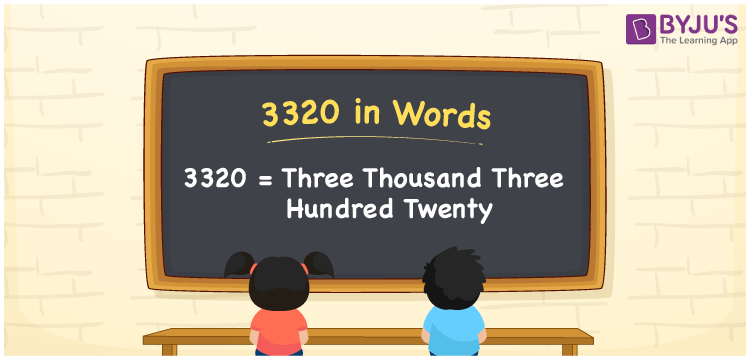# 3320 in Words

3320 in words is written as Three thousand three hundred twenty. In both the International System of Numerals and the Indian System of Numerals, 3320 in words, is written as Three thousand three hundred twenty. The number 3320 is a Cardinal Number as it represents some quantity. For example, “that item costs 3320 rupees”.

 3320 in Words Three thousand three hundred twenty Three thousand three hundred twenty in Number 3320

## 3320 in English Words

3320 in English words is read as “Three thousand three hundred twenty”.## How to Write 3320 in Words?

To write 3320 in words, we shall use the place value chart. In the place value chart, put 3 in the thousands, 3 in the hundreds, 2 in the tens, and 0 in the ones, respectively. Let us make a place value chart to write the number 3320 in words.

 Thousands Hundreds Tens Ones 3 3 2 0

Thus, we can write the expanded form as

3 × Thousand + 3 × Hundred + 2 × Ten + 0 × One

= 3 × 1000 + 3 × 100 + 2 × 10 + 0 × 1

= 3000 + 300 + 20 + 0

= 3320

= Three thousand three hundred twenty.

3320 is a natural number which is the successor of 3319 and the predecessor of 3321.

3320 in words – Three thousand three hundred twenty

• Is 3320 an odd number? – No
• Is 3320 an even number? – Yes
• Is 3320 a perfect square number? – No
• Is 3320 a perfect cube number? – No
• Is 3320 a prime number? – No
• Is 3320 a composite number? – Yes

## Frequently Asked Questions on 3320 in Words

Q1

### How to write 3320 in words?

3320 in words is written as Three thousand three hundred twenty.
Q2

### How to write 3320 in words in the International and Indian System of Numerals?

In both, the system of numerals, 3320 in words, is written as Three thousand three hundred twenty.
Q3

### How to write 3320 in a place value chart?

In the place value chart, write 3 in the thousands, 3 in the hundreds, 2 in the tens, and 0 in the ones, respectively.